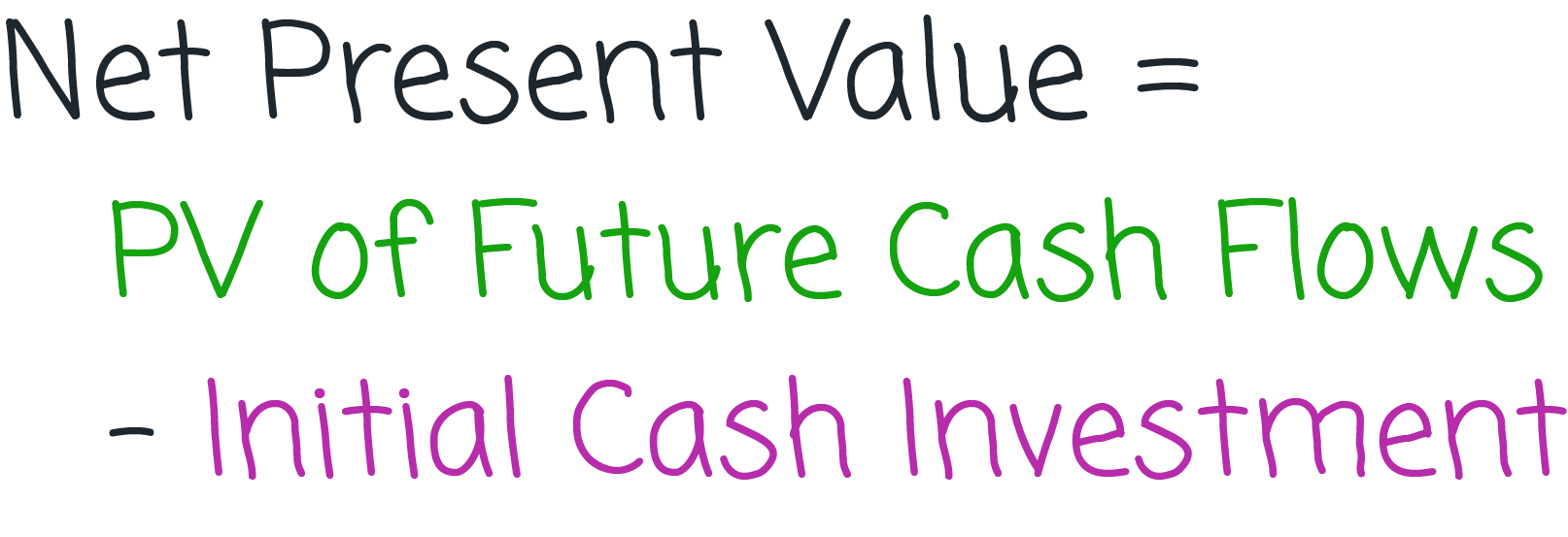# How to Calculate NPV

## Formula & DefinitionIf you read our earlier article of present value and discounted cash flow, then you need to take only a small step to understand net present value (NPV). Recall that you performed a discounted cash flow analysis to find the preset worth of all of the property's future cash flows at a given discount rate.

Let's say that you require a 10.5 % rate of return on your investment. When you discount all of the expected future cash flows back at that rate, the PV that you find as your answer is the amount of cash you need to invest at 10.5 % to achieve those future cash flows - with exactly the same timing and amount that you predicted.

The discount cash flow analysis tells you what the future cash flows are worth at a given rate of return, but that isn't necessarily how much you are going to pay for them. This is where NPV comes in. NPV is the difference between the PV of all future cash flows and the amount of cash you invest to purchase those cash flows. That's why it's called "net" present value.

If you invest exactly what the future cash flows are worth at a given discount rate (rate of return), then your investment is earning exactly that rate. If the PV of the cash flows is greater than the amount of your investment, you have a positive NPV, which is another way of saying that you're really doing better than the specified rate of return because you're getting more than you expected from your investment. If the PV of the cash flows is less than the amount of your investment, you have a negative NPV. That means that you're not doing as well as the specified rate of return.

A term closely related to NPV is profitability index explained in a separate article.

Zilculator helps real estate professionals calculate net present value easily. Never use a spreadsheet again! Analyze your own property or create investment reports for your clients.

• Sales and Rental comps

## How to Calculate Net Present Value (NPV) of an Investment Property

1. Calculate present value of all future cash flows as described in the article here.
2. Determine the total initial cash investment needed to acquire the property.
3. Subtract these two numbers as shown below:4. Calculation of NPV manually is pretty complex. Instead you can use the Microsoft Excel spreadsheet calculator below.